# 机器学习-如何训练数据调整参数让准确率更高？

2019/08/30 16:07

### 背景介绍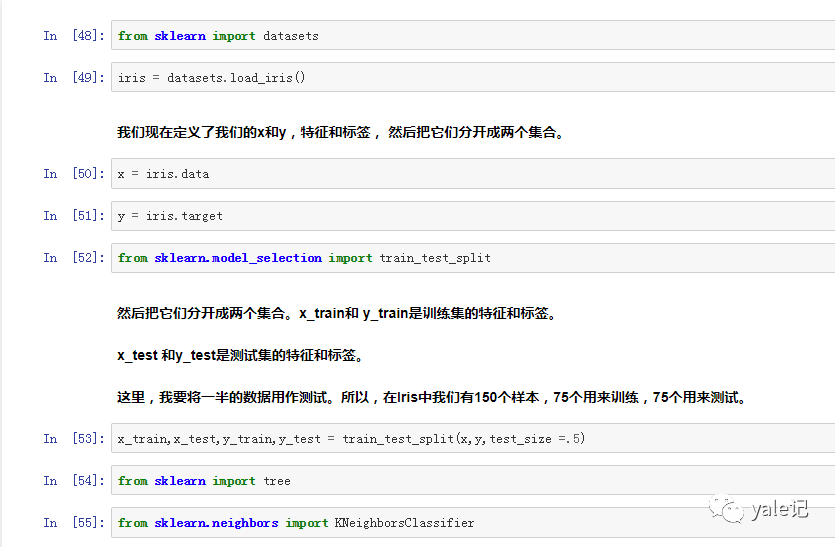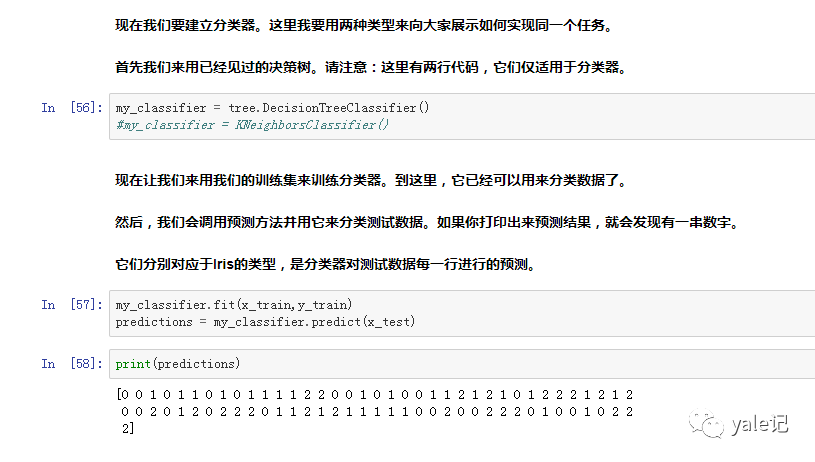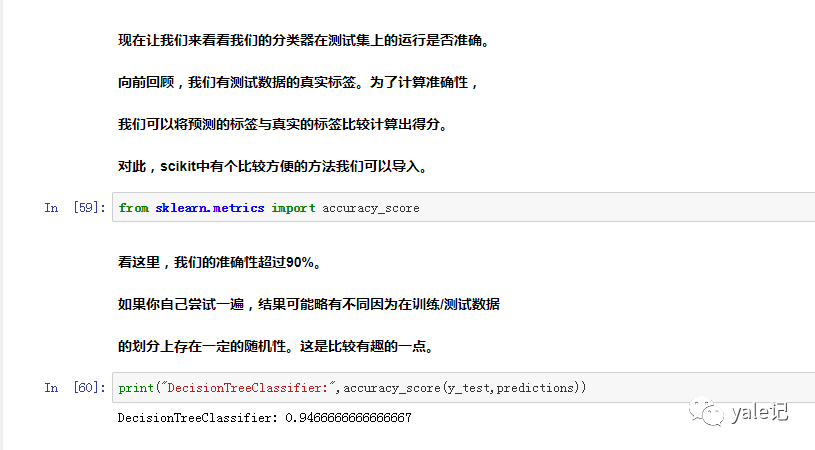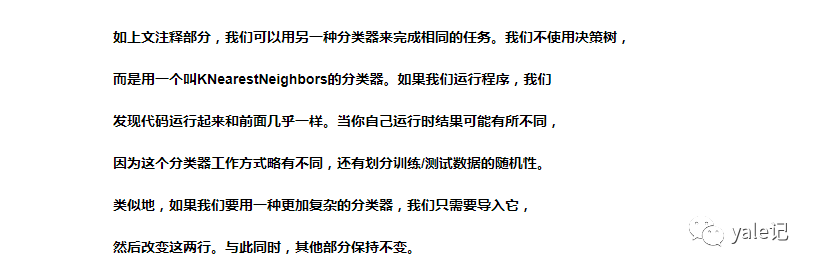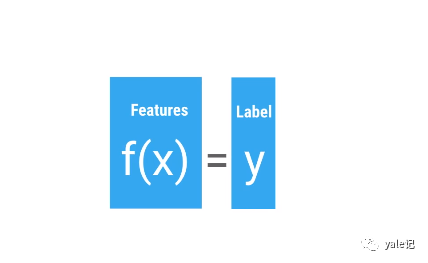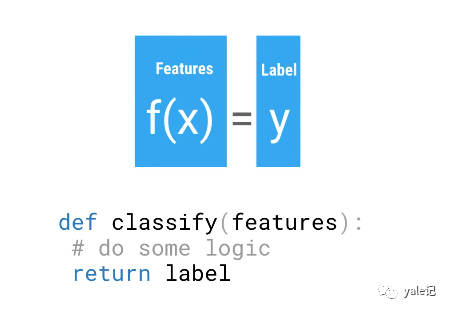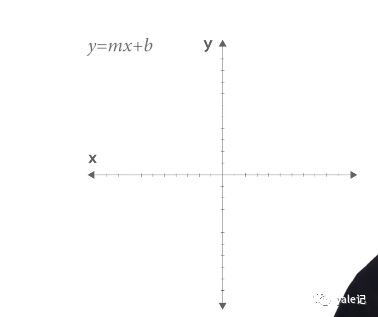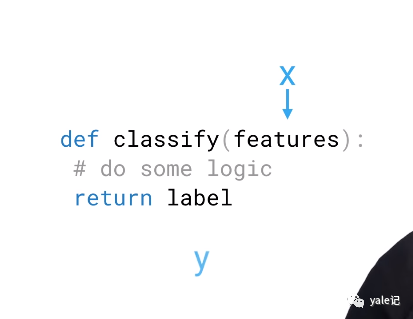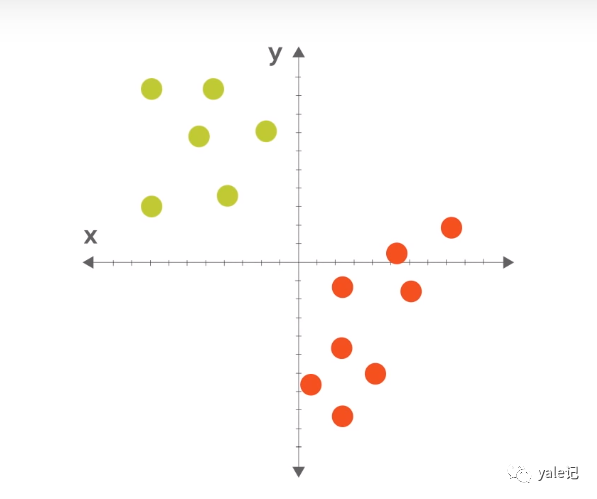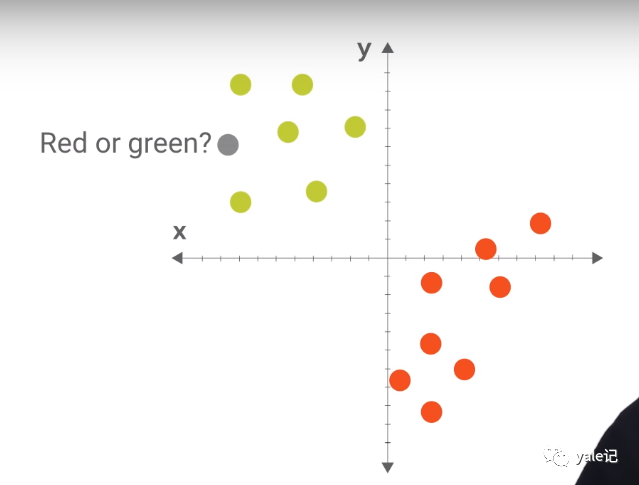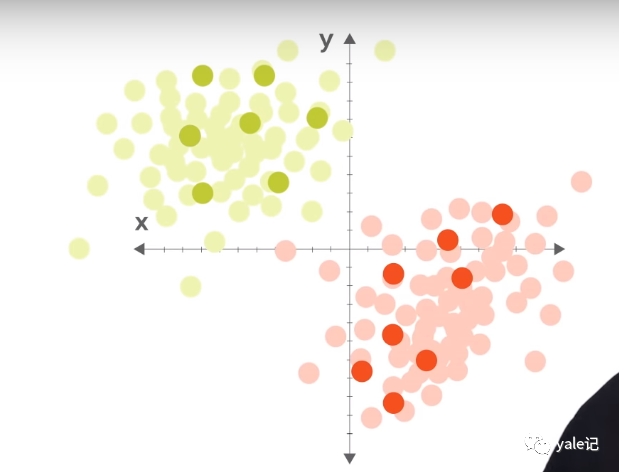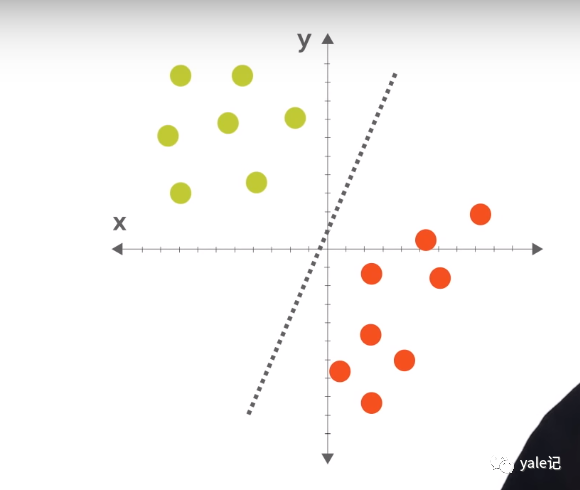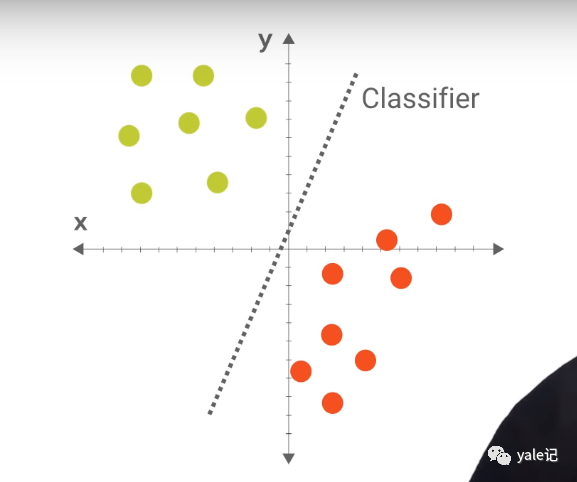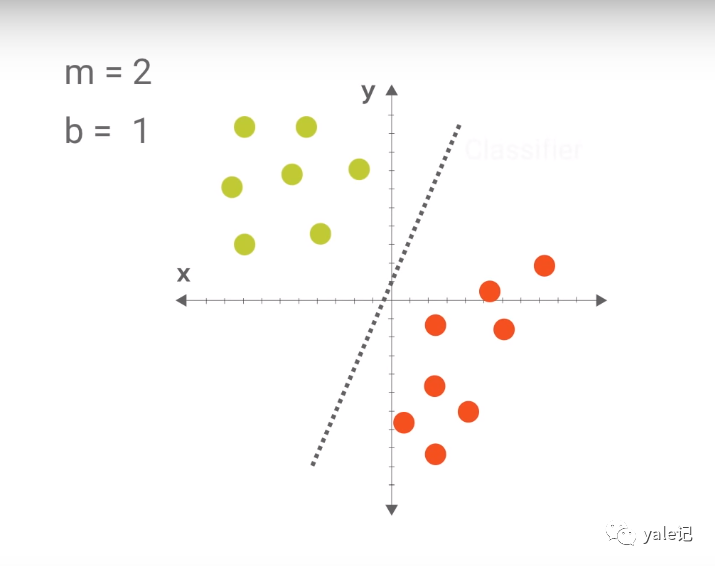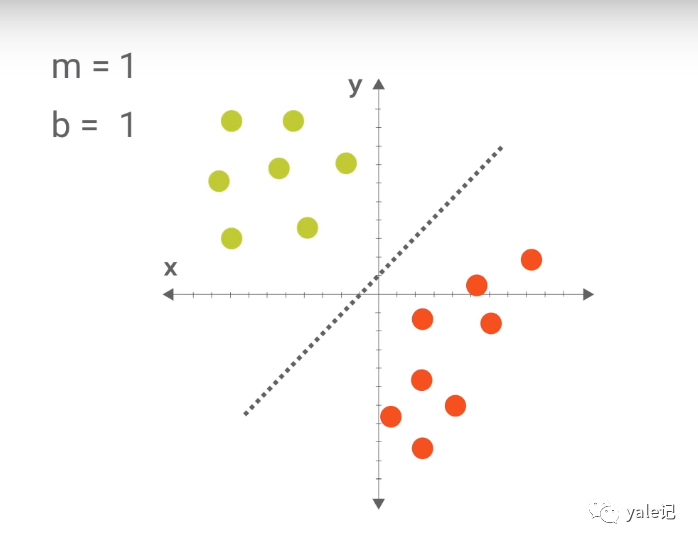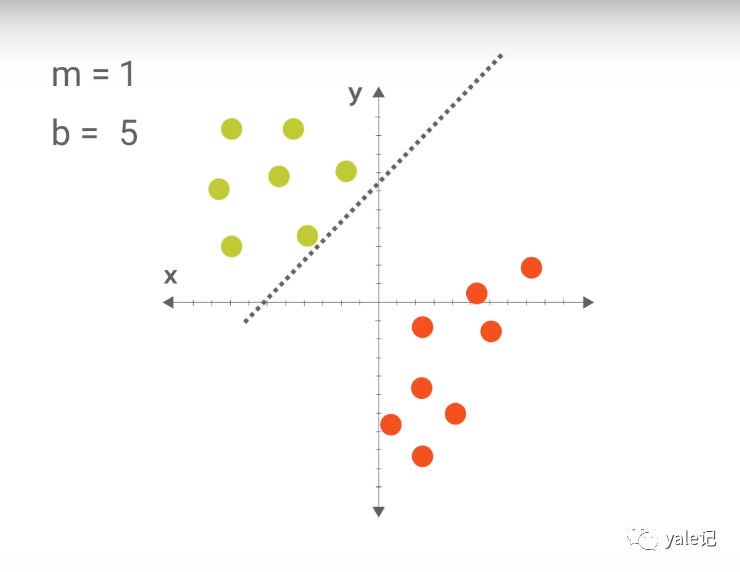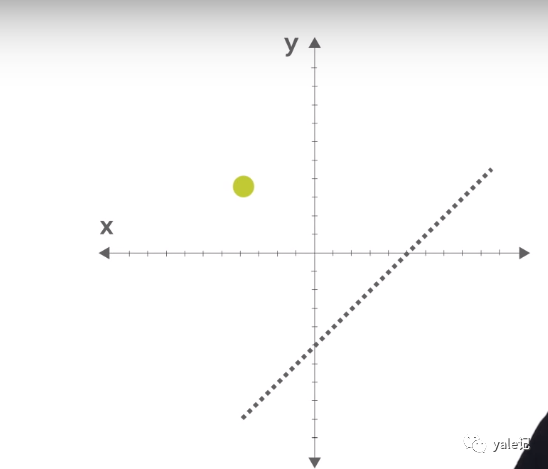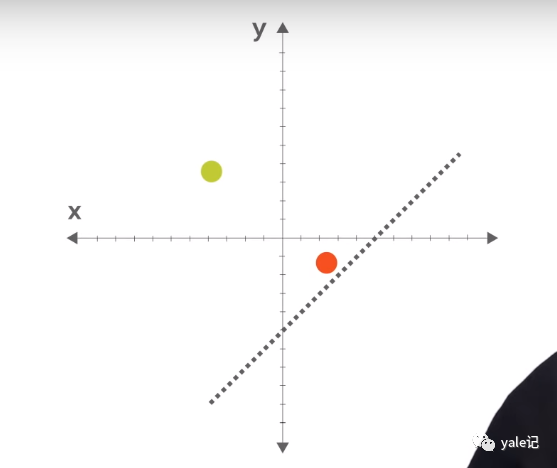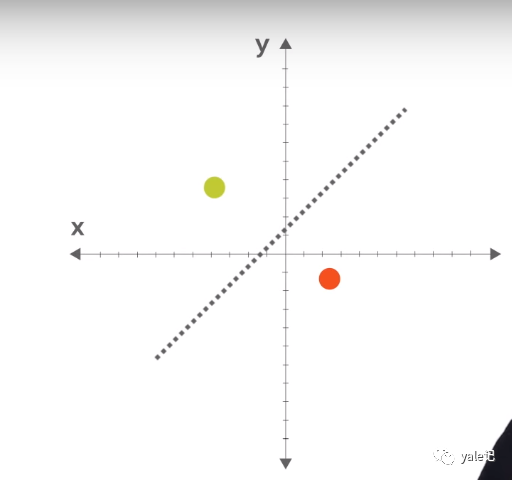#### 示例代码

from sklearn import datasetsiris = datasets.load_iris()x = iris.datay = iris.targetfrom sklearn.model_selection import train_test_splitx_train,x_test,y_train,y_test = train_test_split(x,y,test_size =.5)from sklearn import treefrom sklearn.neighbors import KNeighborsClassifiermy_classifier = tree.DecisionTreeClassifier()#my_classifier = KNeighborsClassifier()my_classifier.fit(x_train,y_train)predictions = my_classifier.predict(x_test)print(predictions)from sklearn.metrics import accuracy_scoreprint("DecisionTreeClassifier:",accuracy_score(y_test,predictions))

### 关注公号0
0 收藏

### 作者的其它热门文章0 评论
0 收藏
0